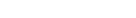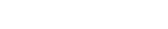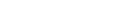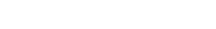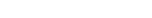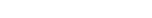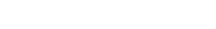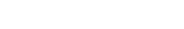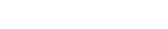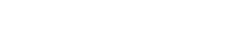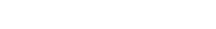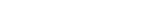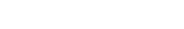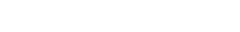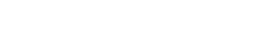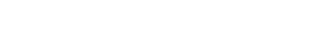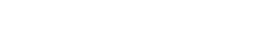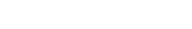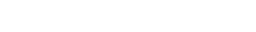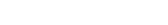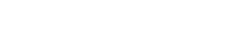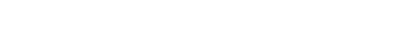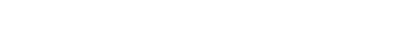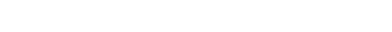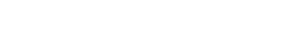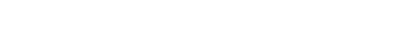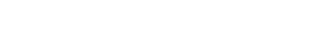xBridgman's thermodynamic equationsEncyclopedia
In thermodynamics
Thermodynamics
Thermodynamics is a physical science that studies the effects on material bodies, and on radiation in regions of space, of transfer of heat and of work done on or by the bodies or radiation...

, Bridgman's thermodynamic equations are a basic set of thermodynamic equations, derived using a method of generating a large number of thermodynamic identities involving a number of thermodynamic quantities. The equations are named after the American physicist Percy Williams Bridgman
Percy Williams Bridgman
Percy Williams Bridgman was an American physicist who won the 1946 Nobel Prize in Physics for his work on the physics of high pressures. He also wrote extensively on the scientific method and on other aspects of the philosophy of science.- Biography :Bridgman entered Harvard University in 1900,...

Exact differential
A mathematical differential is said to be exact, as contrasted with an inexact differential, if it is of the form dQ, for some differentiable function Q....

article for general differential relationships).

The extensive variables of the system are fundamental. Only the entropy
Entropy
Entropy is a thermodynamic property that can be used to determine the energy available for useful work in a thermodynamic process, such as in energy conversion devices, engines, or machines. Such devices can only be driven by convertible energy, and have a theoretical maximum efficiency when...

S , the volume V  and the four most common thermodynamic potentials will be considered. The four most common thermodynamic potentials are:
 Internal energyInternal energyIn thermodynamics, the internal energy is the total energy contained by a thermodynamic system. It is the energy needed to create the system, but excludes the energy to displace the system's surroundings, any energy associated with a move as a whole, or due to external force fields. Internal... U EnthalpyEnthalpyEnthalpy is a measure of the total energy of a thermodynamic system. It includes the internal energy, which is the energy required to create a system, and the amount of energy required to make room for it by displacing its environment and establishing its volume and pressure.Enthalpy is a... H Helmholtz free energyHelmholtz free energyIn thermodynamics, the Helmholtz free energy is a thermodynamic potential that measures the “useful” work obtainable from a closed thermodynamic system at a constant temperature and volume... A Gibbs free energyGibbs free energyIn thermodynamics, the Gibbs free energy is a thermodynamic potential that measures the "useful" or process-initiating work obtainable from a thermodynamic system at a constant temperature and pressure... G

The first derivatives of the internal energy with respect to its (extensive) natural variables S  and V  yields the intensive parameters of the system - The pressure
Pressure
Pressure is the force per unit area applied in a direction perpendicular to the surface of an object. Gauge pressure is the pressure relative to the local atmospheric or ambient pressure.- Definition :...

P  and the temperature
Temperature
Temperature is a physical property of matter that quantitatively expresses the common notions of hot and cold. Objects of low temperature are cold, while various degrees of higher temperatures are referred to as warm or hot...

T . For a simple system in which the particle number
Particle number
The particle number of a thermodynamic system, conventionally indicated with the letter N, is the number of constituent particles in that system. The particle number is a fundamental parameter in thermodynamics which is conjugate to the chemical potential. Unlike most physical quantities, particle...

s are constant, the second derivatives of the thermodynamic potentials can all be expressed in terms of only three material properties
Material properties (thermodynamics)
The thermodynamic properties of materials are intensive thermodynamic parameters which are specific to a given material. Each is directly related to a second order differential of a thermodynamic potential...

 heat capacity (constant pressure)Heat capacityHeat capacity , or thermal capacity, is the measurable physical quantity that characterizes the amount of heat required to change a substance's temperature by a given amount... CP Coefficient of thermal expansionThermal expansionThermal expansion is the tendency of matter to change in volume in response to a change in temperature.When a substance is heated, its particles begin moving more and thus usually maintain a greater average separation. Materials which contract with increasing temperature are rare; this effect is... α Isothermal compressibility βT

Bridgman's equations are a series of relationships between all of the above quantities.

## Introduction

Many thermodynamic equations are expressed in terms of partial derivatives. For example, the expression for the heat capacity at constant pressure is: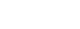which is the partial derivative of the enthalpy with respect to temperature while holding pressure constant. We may write this equation as: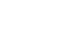This method of rewriting the partial derivative was described by Bridgman (and also Lewis & Randall), and allows the use of the following collection of expressions to express many thermodynamic equations. For example from the equations below we have: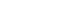and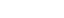Dividing, we recover the proper expression for CP.

The following summary restates various partial terms in terms of the thermodyamic potentials, the state parameters S, T, P, V, and the following three material properties
Material properties (thermodynamics)
The thermodynamic properties of materials are intensive thermodynamic parameters which are specific to a given material. Each is directly related to a second order differential of a thermodynamic potential...

which are easily measured experimentally.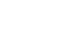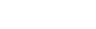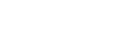## Bridgman's thermodynamic equations

Note that Lewis and Randall use F and E for the Gibbs energy and internal energy, respectively, rather than G and U which are used in this article.